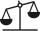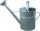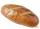# Two bricks

One brick weighs 1kg and half a brick, how many weigh two bricks?

Result

m =  4 kg

#### Solution:Leave us a comment of example and its solution (i.e. if it is still somewhat unclear...):

Showing 0 comments:Be the first to comment!#### To solve this example are needed these knowledge from mathematics:

Do you have a linear equation or system of equations and looking for its solution? Or do you have quadratic equation? Most natural application of trigonometry and trigonometric functions is a calculation of the triangles. Common and less common calculations of different types of triangles offers our triangle calculator. Word trigonometry comes from Greek and literally means triangle calculation.

## Next similar examples:

1. Brick weightHalf the weight of a brick plus 20 pounds is equal to 1/3 the weight of the brick plus 30 pounds. How much does the brick weigh?
2. BrickIsosceles scale has on one side all brick and second weight 1 kg and 1/4 of brick. The balance is in equilibrium. What is the weight of a brick?
3. CanWatering can full of water weighs 10 kg. Half-full can weighs 5.5 kg. How much weigh can?
4. Bread5 of the same bread has the same weight as three bread and 4 kg of fruit. What weight has one bread?
5. Forest nurseryIn the forest nursery after winter, they found that 1/10 stems died out of them. For them, they land 193 new spruces. How many spruces are in the forest nursery?
6. NormThree workers planted 3555 seedlings of tomatoes in one dey. First worked at the standard norm, the second planted 120 seedlings more and the third 135 seedlings more than the first worker. How many seedlings were standard norm?
7. Simple equation 5Solve equation with fractions: X × 3/8 = 1/2
8. Negative in equation2x + 3 + 7x = – 24, what is the value of x?
9. Find xSolve: if 2(x-1)=14, then x= (solve an equation with one unknown)
10. Unknown number 11That number increased by three equals three times itself?
11. Simple equationSolve the following simple equation: 2. (4x + 3) = 2-5. (1-x)
12. Equation 29Solve next equation: 2 ( 2x + 3 ) = 8 ( 1 - x) -5 ( x -2 )
13. Equation 20In given equation: 8/9-4/5=2/9+x, find x
14. EquatiomSolve equation with negatives: X/(-5) + 2 = -9
15. Eq1Solve equation: 4(a-3)=3(2a-5)
16. If-then equationIf 5x - 17 = -x + 7, then x =
17. Dropped sheetsThree consecutive sheets dropped from the book. The sum of the numbers on the pages of the dropped sheets is 273. What number has the last page of the dropped sheets?